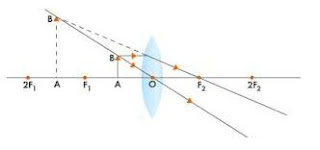## Solutions of Refraction of Light Lakhmir Singh Manjit Kaur VSAQ, SAQ, MCQ, HOTS, and LAQ Pg No. 227 Class 10 Physics

1. What name is given to the ratio of sine of angle of incidence to the sine of angle of refraction?

→ The ratio of sine of angle of incidence to the sine of angle of refraction is called Refractive index.

2. Write the relation between the angle of incidence and the angle of refraction for a medium.

→ The relation between the angle of incidence and the angle of refraction for a medium is3. What is the unit of refractive index?

→ None

4. Which has higher refractive index: water or glass?

→ Glass.

5. Refractive indices of carbon disulphide and ethyl alcohol are 1.63 and 1.36 respectively. Which is optically denser?

→ Refractive indices of carbon disulphide = 1.63
Refractive indices of ethyl alcohol = 1.36
Thus, Carbon disulphide is more denser than the ethyl alcohol.

6. The refractive index of diamond is 2.42. What is the meaning of this statement in relation to the speed of light?

→ Refractive index of diamond = 2.42
Since, the relation between the angle of incidence and the angle of refraction for a medium isThis means that the ratio of the speed of light in air to the speed of light in diamond is equal to 2.42.

7. If the refractive index for light going from air to diamond be 2.42, what will be the refractive index for light going from diamond to air?

→ The relation between the angle of incidence and the angle of refraction for a medium isThus, refractive index for light going from diamond to air = 0.41.

8. How is the refractive index of a material related to the speed of light in it?

→ The relation between the angle of incidence and the angle of refraction for a medium is9. State whether it is true or not:
When a ray of light goes from air into a clear material, you see the ray bend. How much the ray bends is determined by the Refractive index of the material.

→ True.

10. Give three examples of materials that refract light rays. What happens to the speed of light rays when they enter these materials?

→ Water, glass and diamond.
When light rays enter these materials, their speed decreases.

11. Define Snell's law of refraction. A ray of light is incident on a glass slab at an angle of incidence of 60°. If the angle of refraction be 32.7°, calculate the refractive index of glass. (Given: sin 60° = 0.866 and sin 30° = 0.5).

→ According to the Snell's law of refraction, the ratio of sine of angle of incidence to the sine of angle of refraction is constant for a given pair of media.Given: Angle of incidence = 60°
Angle of refraction = 30°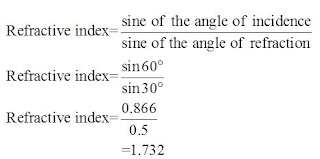12. The speed of light in vacuum and in two different glasses is given in the table below:(a) Calculate the absolute refractive indexes of flint glass and crown glass.
(b) Calculate the relative refractive index for light going from crown glass to flint glass.

→ The relation between the angle of incidence and the angle of refraction for a medium is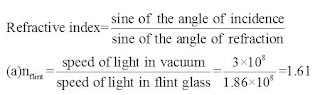Refractive indexes of flint glass = 1.61Refractive indexes of crown glass = 1.52The relative refractive index for light going from crown glass to flint glass = 1.059

13. The speed of light in air is 3 x 108 m/s. In medium X its speed is 2 x 108 m/s and in medium Y the speed of light is 2.5 x 108 m/s. Calculate:
(a) airnx = ?
(b) airny = ?
(c) any=?

→ Given:
Speed of light in air = 3.0x108m/s
Speed of light in medium X = 2.0x108m/s
Speed of light in medium Y = 2.50x108m/s
(a) airnx = ?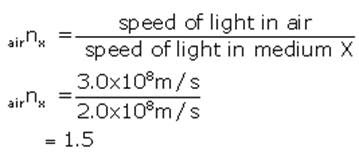(b) airny = ?(c) xny = ?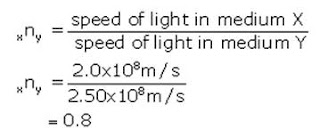14. What is the speed of light in a medium of refractive index 1.4 if its speed in air is 3, 00,000 km/s?

→ Refractive index of medium = 1.4
Speed of light in air = 3,00,000km/s
We know that15. The refractive index of glass is 1.7. Calculate the speed of light in glass. The speed of light in air is 3.0 x 108 ms-1

→ Given
Refractive index of glass = 1.7
Speed of light in air = 3.0x108m/s
We know that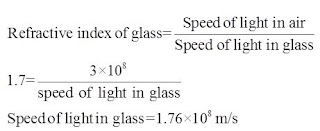16. The speed of light in water is 2.25 x 108 m/s. If the speed of light in vacuum be 3 x 108 m/s, calculate the refractive index of water.

→ Speed of light in vacuum = 3.0 x 108m/s
Speed of light in water = 2.25 x 108m/s
Refractive index of water = ?
We know that17. Light enters from air into diamond which has a refractive index of 2.42. Calculate the speed of light in diamond. The speed of light in air is 3.0 x108 ms-1.

→ Given
Refractive index of diamond = 2.42
Speed of light in air = 3.0x108m/s
We know that18A. State and explain the laws of refraction of light with the help of a labeled diagram.

→ Laws of refraction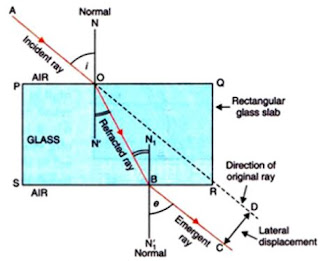First law of refraction states that, the incident ray, the refracted ray and the normal at the point of incidence, all lie in the same plane.

Second law of refraction states that, the ratio of the sine of angle of incidence to the sine of angle of refraction is constant for a given pair of media.

18B. What is meant by the refractive index of a substance?

→ The relation between the angle of incidence and the angle of refraction for a medium is known as refractive index of that medium.18C. Light travels through air at 300 million ms-1. On entering water it slows down to 225 million ms-1. Calculate the refractive index of water.

→ Speed of light in air = 3 x 108 m/sec
Speed of light in water = 2.25 x 108 m/sec
We know that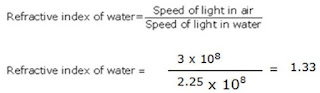Multiple Choice Questions (MCQs)-Pg-228

19. The refractive indices of four substances P, Q, Rand S are 1.50, 1.36, 1.77 and 1.31 respectively. The speed of light is the maximum in the substance:
A. P
B. Q
C. R
D. S20. The refractive indices of four materials A, B, C and D are 1.03, 1.13, 1.92 and 1.72 respectively. When the light rays pass from air into these materials, they refract the maximum in:
A. Material A
B. material B
C. Material C
D. material D

→ Refraction is maximum in the material with maximum refractive index.

21. The refractive index of glass for light going from air to glass is . The refractive index for light going from glass to air will be:
A. 1/3
B. 4/5
C. 4/6
D. 5/2

→ The refractive index for light going from glass to air = 1/ refractive index of glass for light going from air to glass = 2/3

22. The refractive indices of four media A, B, C and Dare 1.44, 1.52, 1.65 and 1.36 respectively. When light travelling in air is incident in these media at equal angles, the angle of refraction will be the minimum:
A. In medium A
B. in medium B
C. In medium C
D. in medium D

→ The angle of reflection is minimum in the medium with more refractive index.

23. The speed of light in substance X is 1.25 x 108 m/s and that in air is 3 x 108 m/s. The refractive index of this substance will be:
A. 2.4
B. 0.4
C. 4.2
D. 3.75

→ Speed of light in substance X is 1.25 x 108 m/s
Speed of light in substance air is 3 x 108 m/s
The refractive index of this substance will be speed of light in substance air/ speed of light in substance X.

24. The refractive indexes of four substances P, Q, R and S are 1.57, 1.54, 2.52 and 1.33 respectively. When light travelling in air is incident on these substances at equal angles, the angle of refraction will be the maximum in:
A. Substance P
B. substance Q
C. Substance R
D. substance S

→ The angle of refraction will be the maximum in substance with minimum refractive index.

25. The refractive index of water is:
A. 1.33
B. 1.50
C. 2.42
D. 1.36

→ The refractive index of water is 1.33.

26. The refractive index of water with respect to air is 4/3. The refractive index of air with respect to water will be:
A. 1.75
B. 0.50
C. 0.75
D. 0.25

→ The refractive index of air with respect to water = 1/ refractive index of water with respect to air.

27. Refractive indices of water, sulphuric acid, glass and carbon disulphide are 1.33, 1.43, 1.53 and 1.63 respectively. The light travels slowest in:
A. Sulphuric acid
B. glass
C. mithaen
D. carbon disulphide

→ The light travels slowest in material with maximum refractive index.

28. The refractive index of glass with respect to air is 3/2 and the refractive index of water with respect to air is 4/3. The refractive index of glass with respect to water will be
A. 1.525
B. 1.225
C. 1.425
D. 1.125

→ Refractive index of glass with respect to air is 3/2
The refractive index of water with respect to air is 4/3
Refractive index of glass with respect to water will be 3/4 x 3/2 = 9/8 = 1.125

Questions Based on High Order Thinking Skills (HOTS)-Pg-229

29. The following table gives the refractive indices of a few media:Use this table to give an example of:
(i) a medium pair so that light speeds up when it goes from one of these medium to another.
(ii) A medium pair so that light slows down when it goes from one of these medium to another.

→ (i) Example of a medium pair so that light speeds up when it goes from one of these medium to another is Crown glass to water.
(ii) Example of A medium pair so that light slows down when it goes from one of these medium to another is Water to diamond.

30. Refractive indices of four media A, B, C and Dare given below: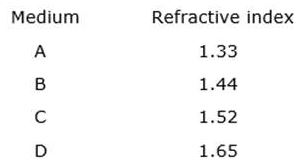In which of these four media is the speed of light (i) maximum, and (ii) minimum ?

→ (i) Speed of light is maximum in A as it has least refractive index.
(ii) Speed of light is minimum in D as it has highest refractive index.

1. Name the lens which can concentrate sun's rays to a point and bum a hole in a piece of paper.

→ Converging lens is the lens which can concentrate sun's rays to a point and bum a hole in a piece of paper.

2. Give the usual name for the following :
A point inside a lens through which the light passes undeviated.

→ A point inside a lens through which the light passes undeviated is called Optical centre.

3. A 1 cm high object is placed at a distance of 2f from a convex lens. What is the height of the image formed?

→ The height of the image formed is same as the height of the object that is 1 cm.

4. If the image formed by a convex lens is of the same size as that of the object, what is the position of the image with respect to the lens?

→ The image formed by a convex lens is of the same size as that of the object At 2F.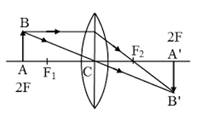5. If an object is placed at the focus of a convex lens, where is the image formed?

→ The image is formed at infinity.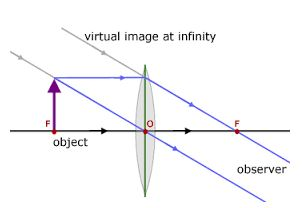6. Where should an object be placed in order to use a convex lens as a magnifying glass?

→ Object should be placed at a distance less than focal length order to use a convex lens as a magnifying glass.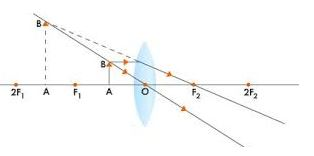7. Where should an object be placed in front of a convex lens so as to obtain its virtual, erect and magnified image?

→ The object should be placed within focus to obtain its virtual, erect and magnified image.8. Where an object should be placed in front of a convex lens so as to obtain its real, inverted and magnified image?

→ Object should be placed between f and 2f in front of a convex lens so as to obtain its real, inverted and magnified image.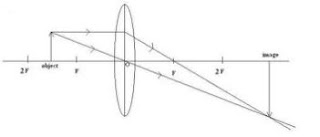9. For what position of an object a real, diminished image is formed by a convex lens?

→ Beyond 2F of an object a real, diminished image is formed by a convex lens.10. If an object is at a considerable distance (or infinity) in front of a convex lens, where is the image formed?

→ If an object is at a considerable distance (or infinity) in front of a convex lens, the image is formed at focus F.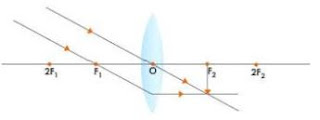11. Draw the given diagram in your answer book and complete it for the path of a ray of light after passing through the lens.12. What type of lens would you use as a magnifying glass? How close must the object be to the lens?

→ Convex lens would be used as a magnifying glass
The object must be within the focus of the lens.13. Name two factors on which the focal length of a lens depends.

→ Focal length of a lens depends on the
1) refractive index of the glass
2) the curvature of its two surfaces.

14. State any two uses of convex lenses.

→ Two uses of convex lenses are that it can be used as a magnifying glass and for making a simple camera.

15. Fill in the following blanks with suitable words:
(a) _______ rays of light are refracted by a convex lens to a point called the focus.
(b) The image in a convex lens depends upon the distance of the object from the______.

→ a) Parallel
b) lens

16. What is a lens? Distinguish between a convex lens and a concave lens. Which of the two is a converging lens: convex lens or concave lens?

→ A lens is a piece of transparent glass bound by two spherical surfaces..Convex lens is a converging lens.

17A. Explain with the help of a diagram, why the convex lens is also called a converging lens.

→ A convex lens also known as converging type because it converges a parallel beam of light rays passing through it.17B. Define principal axis, principal focus and focal length of a convex lens.

→ The principal axis of a lens is a line passing through the optical centre of the lens and perpendicular to both the faces of the lens.
The principal focus of a convex lens is a point on its principal axis to which light rays parallel to the principal axis converge after passing through the lens.
The focal length is the distance of the principle focus from the optical center of a lens.

18A. Explain with the help of a diagram, why the concave lens is also called a diverging lens.

→ A concave lens is known as diverging lens because it diverges the parallel rays of light passing through it.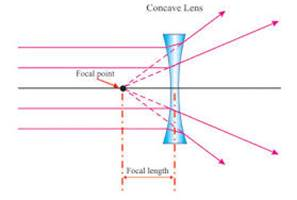18B. Define the principal focus of a concave lens.

→ The principal focus of a concave lens is a point on its principal axis from which parallal light rays appear to diverge after passing through the lens.

19. Draw a ray diagram to show the formation of a real magnified image by a convex lens. (In your sketch the position of object and image with respect to the principal focus of lens should be shown clearly).

→ The formation of a real magnified image by a convex lens.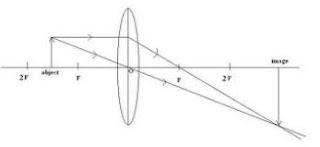20. Describe with the help of a ray-diagram, the formation of image of a finite object placed in front of a convex lens between f and 2f Give two characteristics of the image so formed.

→ If object is placed in between f and 2f, the image will form on the other side of the lens beyond 2f as shown below.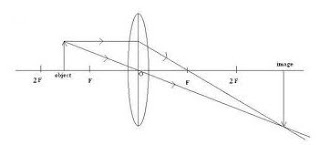Characteristics of image formed is
a) real and inverted.
b) magnified.

21. Describe with the help of a ray diagram the nature, size and position of the image formed when an object is placed in front of a convex lens between focus and optical center. State three characteristics of the image formed.

→ In the diagram, the object is placed in front of a convex lens between focus and optical centre. The image is formed on the same side as the object as shown below.Characteristics of image formed is
a. virtual and erect.
b. larger than the object
c. formed behind the object.

22. An object is placed at a distance equal to 2f in front of a convex lens. Draw a labeled ray diagram to show the formation of image. State two characteristics of the image formed.

→ An object is placed at a distance equal to 2f in front of a convex lens. The ray diagram is as suchCharacteristic of image formed is
a. real and inverted.
b. same size as the object.

23. Describe with the help of a ray-diagram, the size, nature and position of the image formed by a convex lens when an object is placed beyond 2f in front of the lens.

→ The size, nature and position of the image formed by a convex lens when an object is placed beyond 2f in front of the lens is real, inverted and smaller than the object.24. Describe with the help of a ray diagram the nature, size and position of the image formed when an object is placed at infinity (considerable distance) in front of a convex lens. State three characteristics of the image so formed.

→ The nature, size and position of the image formed when an object is placed at infinity (considerable distance) in front of a convex lens is
a) real.
b) inverted.
c) highly diminished.25A. What type of lens is shown in the diagram on the right ? What will happen to the parallel rays of light? Show by completing the ray diagram.

→ The lens shown in convex. the parallel rays will converge to a point called focus (F).25B. Your eye contains a convex lens. Why is it unwise to look at the sun?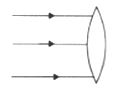→ It is unwise to look at the sun because the convex lens focusses a lot of sun rays into our eyes and this may damage the eyes.

26. Where the object must be placed for the image formed by a converging lens to be:
(a) Real, inverted and smaller than the object?
(b) Real, inverted and same size as the object?
(c) Real, inverted and larger than the object?
(D) Virtual, upright and larger than the object?

→ a) for the image formed by a converging lens to be Real, inverted and smaller than the object Beyond 2F.b) for the image formed by a converging lens to be Real, inverted and same size than the object At 2F.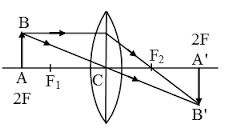c) for the image formed by a converging lens to be Real, inverted and larger than the object Between F and 2F.d) for the image formed by a converging lens to be Virtual, upright and larger than the object Between F and optical centre.27. Draw a diagram to show how a converging lens held close to the eye acts as a magnifying glass. Why is it usual to choose a lens of short focal length for this purpose rather than one of long focal length?